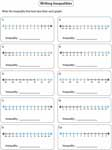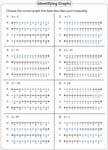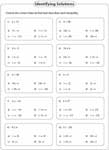1. Worksheets>
2. Math>
3. Algebra>
4. Inequalities

# Solving and Graphing Inequalities Worksheets

Linear inequality worksheets contain graphing inequalities, writing inequality from the graph, solving one-step, two-step and multi-step inequalities, graphing solutions, solving and graphing compound inequalities, absolute value inequalities and more.

One-Step Inequalities Worksheets

Translate verbal phrases into algebraic expressions, solve one-step inequalities, identify solutions for inequalities and more.

Two-Step Inequalities Worksheets

Interpret verbal phrases as algebraic expressions, identify values that satisfy an inequality and solve inequalities as well.

Multi-Step Inequalities Worksheets

Multi-step inequalities worksheets contain solving, graphing, identifying solutions, solutions in interval notation.

Compound Inequalities Worksheets

This page provides educational supplement to practice solving and graphing compound inequalities. More than 50 worksheets included.

Graphing Linear Inequalities Worksheets

Explore this compilation of worksheets to graph the linear inequality, write inequality of the graph, complete the inequality and more.

Absolute Value Inequalities Worksheets

Solve the absolute value inequalities and graph the solution using the number lines. Interval notation worksheets are included too.

Solve quadratic inequalities algebraically, using the graph and table format with these quadratic inequalities worksheets.

Inequalities Worksheets

## Inequalities Worksheets for Beginners

Translating Phrases

Translate each phrase into algebraic inequality. There are 16 problems in each worksheet.Picking out the solutions

For each inequality, circle all the possible values that satisfy the expression.Graphing Inequality using a Number Line

Each worksheet has 12 problems graphing basic inequalities. Moderate level has numberings in different increments.Easy:

Moderate:

Writing Inequalities

Write the inequality that best describes each graph.Easy:

Moderate:

Select the Correct Graph

Choose the correct graph for each inequality in a single variable.Identifying Inequalities

Each worksheet has eight questions identifying inequality expression for each graph.Solutions in Interval Notation

Choose the correct interval notation that best describes each inequality.